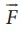Home | | Physics 11th std | Solved Example Problems for Gravitational potential V(r)

# Solved Example Problems for Gravitational potential V(r)

Water falls from the top of a hill to the ground. Why?

### EXAMPLE 6.5

Water falls from the top of a hill to the ground. Why?

This is because the top of the hill is a point of higher gravitational potential than the surface of the Earth i.e.Vhill > VgroundThe motion of particles can be analyzed more easily using scalars like U ( r )or V(r)  than vector quantities likeor. In modern theories of physics, the concept of potential plays a vital role.

### EXAMPLE 6.6

Consider four masses m1, m2, m3, and m4 arranged on the circumference of a circle as shown in figure belowCalculate

a) The gravitational potential energy of the system of 4 masses shown in figure.

b) The gravitational potential at the point O due to all the 4 masses.

Solution

The gravitational potential energy U( r ) can be calculated by finding the sum of gravitational potential energy of each pair of particles.If all the masses are equal, then m1 = m2 = m3 = m4 = MThe gravitational potential V(r) at a point O is equal to the sum of the gravitational potentials due to individual mass. Since potential is a scalar, the net potential at point O is the algebraic sum of potentials due to each mass.Study Material, Lecturing Notes, Assignment, Reference, Wiki description explanation, brief detail
11th Physics : UNIT 6 : Gravitation : Solved Example Problems for Gravitational potential V(r) |